﻿ Multiplication and Division Tables (Level 3) - Word Problems

• A. 7
• B. 24
• C. 32
• D. 12

• A. 132
• B. 23
• C. 144
• D. 34

• A. 21
• B. 4
• C. 10
• D. 15

• A. 24
• B. 10
• C. 2
• D. 12

• A. 11
• B. 72
• C. 60
• D. 36

# More by Benjé Deacon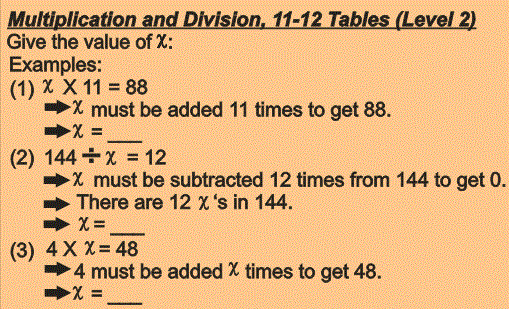##### Multiplication and Division Tables: 11-12 Tables (...

Multiplication and Division tables – 11 and 12 Tables (Level 2). This quiz contains 144 questions. There is an ϰ involved in every question of which the correct value must be given in the answer. Examples: (1) 2 X ϰ = 22  ⇒ 2 must be added ϰ times to get 22 ⇒ ϰ = ___ (2) ϰ ÷ 12 = 3 ⇒ 12 must be subtracted 3 times from ϰ to get 0 ⇒ There are 3 12’s in x ⇒ ϰ = ___ The general question for this quiz is: Give the correct value of ϰ. This quiz will improve your knowledge of the 11-12 Multiplication and Division Tables. .

\$4.00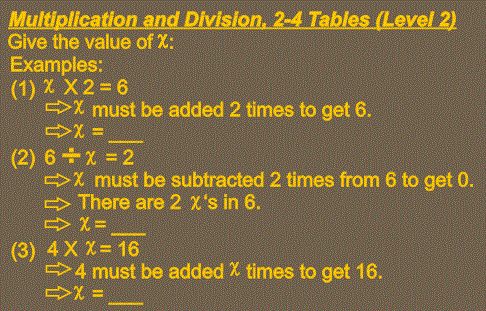##### Multiplication and Division Tables: 2-4 Tables (Le...

Multiplication and Division tables – 2 to 4 Tables (Level 2). This quiz contains 216 questions. There is an ϰ involved in every question of which the correct value must be given in the answer. Examples: (1) 2 X ϰ = 6  ⇒ 2 must be added ϰ times to get 6 ⇒ ϰ = ___ (2) ϰ ÷ 4 = 5 ⇒ 4 must be subtracted 5 times from ϰ to get 0 ⇒ There are 5 4’s in x ⇒ ϰ = ___ The general question for this quiz is: Give the correct value of ϰ. This quiz will improve your knowledge of the 2-4 Multiplication and Division Tables.

\$6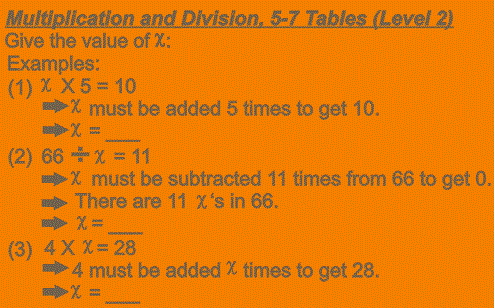##### Multiplication and Division Tables: 5-7 Tables (Le...

Multiplication and Division tables – 5 to 7 Tables (Level 2). This quiz contains 216 questions. There is an ϰ involved in every question of which the correct value must be given in the answer. Examples: (1) 2 X ϰ = 10  ⇒ 2 must be added ϰ times to get 10 ⇒ ϰ = ___ (2) ϰ ÷ 7 = 5 ⇒ 7 must be subtracted 5 times from ϰ to get 0 ⇒ There are 5 7’s in x ⇒ ϰ = ___ The general question for this quiz is: Give the correct value of ϰ. This quiz will improve your knowledge of the 5-7 Multiplication and Division Tables.

\$6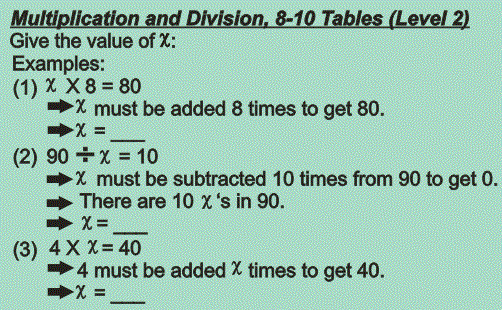##### Multiplication and Division Tables: 8-10 Tables (L...

Multiplication and Division tables – 8 to 10 Tables (Level 2). This quiz contains 216 questions. There is an ϰ involved in every question of which the correct value must be given in the answer. Examples: (1) 2 X ϰ = 16  ⇒ 2 must be added ϰ times to get 16 ⇒ ϰ = ___ (2) ϰ ÷ 9 = 5 ⇒ 9 must be subtracted 5 times from ϰ to get 0 ⇒ There are 5 9’s in x ⇒ ϰ = ___ The general question for this quiz is: Give the correct value of ϰ. This quiz will improve your knowledge of the 8-10 Multiplication and Division Tables.

\$6.00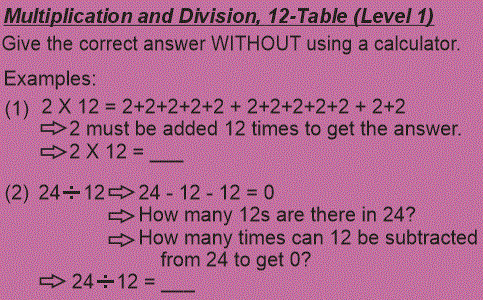##### Multiplication and Division Tables: 12-Table (Leve...

Give the correct answer WITHOUT using a calculator.

\$2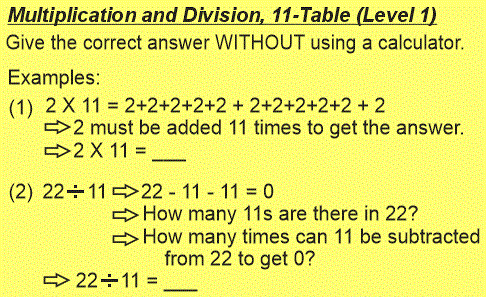##### Multiplication and Division Tables: 11-Table (Leve...

Give the correct answer WITHOUT using a calculator.

\$2